# EddyCurrent

Constant loss model under sinusoidal magnetic conditions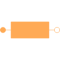# Information

This information is part of the Modelica Standard Library maintained by the Modelica Association.

The eddy current loss model with respect to fundamental wave effects is designed in accordance to FluxTubes.Basic.EddyCurrent and FundamentalWave.Components.EddyCurrent.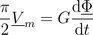.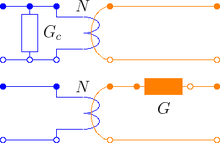Due to the nature of eddy current losses, which can be represented by symmetric conductors in an equivalent electric circuit (Fig. 1), the respective number of phases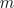has to be taken into account. Assume that theconductances of the equivalent circuit are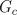, the conductance for the eddy current loss model is determined by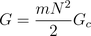where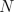is the number of turns of the symmetric electro magnetic coupling.

For such anphase system the relationship between the voltage and current space phasors and the magnetic flux and magnetic potential difference phasor is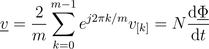,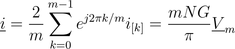,

where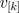and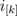are the phase voltages and currents, respectively.

The dissipated loss power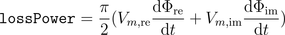can be determined for the space phasor relationship of the voltage and current space phasor.

FluxTubes.Basic.EddyCurrent

# Parameters (3)

useHeatPort Value: false Type: Boolean Description: =true, if heatPort is enabled Value: 273.15 Type: Temperature (K) Description: Fixed device temperature if useHeatPort = false Value: Type: Conductance (S) Description: Equivalent symmetric loss conductance

# Connectors (3)

port_p port_n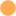Type: PositiveMagneticPort Description: Positive quasi-static magnetic port of fundamental wave machines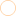Type: NegativeMagneticPort Description: Negative quasi-static magnetic port of fundamental wave machines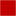Type: HeatPort_a Description: Optional port to which dissipated losses are transported in form of heat

# Components (3)

V_m Phi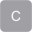Description: Complex magnetic potential differenceType: ComplexMagneticFlux Description: Complex magnetic fluxType: Complex

# Used in Examples (1)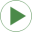EddyCurrentLosses Modelica.Magnetic.QuasiStatic.FundamentalWave.Examples.Components Comparison of equivalent circuits of eddy current loss models

# Used in Components (1)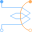SymmetricMultiPhaseWinding Modelica.Magnetic.QuasiStatic.FundamentalWave.BasicMachines.Components Symmetric winding model coupling electrical and magnetic domain